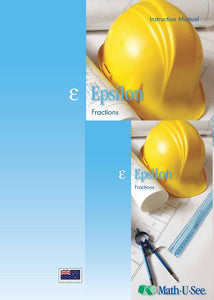or 6 weekly interest-free payments from \$10.83 withwhat's this?Demme Learning

# Math.U.See Epsilon

Regular price \$65.00 \$35.88 Unit price per
Tax included. Shipping calculated at checkout.

## Math.U.See Epsilon

#### Fractions and other topics.

Now that students have learned basic operations with whole numbers, Epsilon covers these same operations with fractions. Fractions are presented in an intuitive way with visual explanations of equivalent fractions, common denominators, and fractions and numbers larger than 1. Fractions and operations are illustrated using our proprietary Fraction Overlay manipulatives.

Major Concepts and Skills Include:

• Recognizing and generating equivalent fractions
• Understanding addition, subtraction, multiplication, and division of fractions and mixed numbers
• Fluently adding, subtracting, multiplying, and dividing fractions and mixed numbers

• Using multiple strategies to recognize common factors
• Understanding grouping symbols and their effect on order of operations
• Interpreting and solving word problems
• Comparing, and converting decimal fractions
• Finding the area and circumference of circles
• Representing fractions and fractional measurements on line plots and number lines
• Using coordinates to represent ordered relationships

Epsilon Instruction Pack includes: Instruction Manual & DVD

Epsilon Student pack includes: Student Workbook & Test & Activity booklet.

Grab an extra Student Pack for an additional Student.

For a complete set to get started with Math.U.See you will need to purchase the the fraction overlay set, the Epsilon instruction pack & Epsilon Student Pack